##### Child pages
• Error: Non-zero value

## Key

• This line was removed.
• Formatting was changed.
HTML Wrap
class section group
HTML
`<span data-swiftype-index="true">`
html
html-wrap
class col span_3_of_5

If you try to record a transaction that would result in a non-zero inventory valuezero units on hand, you will receive a negative inventory balance might receive the following error.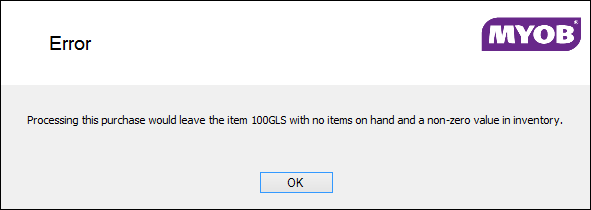Image Added

This means that , while your inventory quantity is will be zero, you still have a positive dollar value attached to the item.

### Why would the average cost be different than the return price?

AccountRight uses the average costing method to track inventory values, which, unlike the first in first out (FIFO) method, calculates the cost of each item in your inventory by using the average cost of the whole group of items in stock.

As the price of the stock you buy changes over time, the average cost of your stock will also change. Problems can occur when your average price doesn't match the actual price of a transaction you're recording. For example, you need to return stock at the original price but it's different to the current average cost. See below for more examples.

UI Expand
title Understanding average cost

AccountRight uses the average costing method of inventory, which values your individual inventory based on the average unit price. The formula for calculating an item's average cost is:

Average Cost = Total Value of the item divided by the Total Quantity of the items

For example, a purchase of 10 items for \$10.00 (tax exclusive) will result in an average cost of \$10.00 per item. If the following day another 10 items were purchased for \$12.00 (tax exclusive), then:

• The total quantity of the items on hand = 20 items.

• The total value of the items = \$ 220.00.

• The average cost of the items = \$11.00 (\$220.00/20)

Complications can sometimes occur if differently priced items are taken out of the inventory and others are returned, while the item's average cost would remain the same.

In the example above, say after the second purchase, 10 items were sold, the 10 remaining items would retain their average cost of \$11.00 (\$110.00 Total Value). If the remaining 10 items were to be returned to the supplier at a price of \$12.00 each (\$120.00 Total Value), then the debit purchase note will result in a:

• zero quantity, and
• a less than zero dollar value.

Because of the less than zero value, the negative inventory error will be displayed. To correct this, an inventory adjustment will first be needed to increase the Total Value of the items. The inventory adjustment will show zero quantity, a zero unit cost and a positive \$10.00 in the amount column.

UI Expand
title Transactions that cause non-zero value errors

You will get the negative inventory balance alert if any of these transactions have occurred:

• An item type purchase debit note (negative purchase) which exceeds either or both the quantity/dollar value of an inventoried item.
• Editing, reversing, or deleting a recorded item purchase which exceeds either or both the quantity/dollar value of an inventoried item.
• Editing, reversing or deleting an item type sale credit note (negative sale). This will remove items from inventory, and as such, the credit note must not remove more quantity/dollar value than is on hand.
• An Inventory Adjustment that attempts to adjust an item's quantity/dollar value to less than zero.
• An Inventory Transfer which attempts to adjust an item's quantity/dollar value to less than zero.
UI Text Box
type tip

Managing a full inventory can sometimes be tricky. The MYOB Inventory Training Course is an excellent resource to better understand AccountRight's inventory and its features - helping you stock smarter.

How can

For example, if you tried to return 7 glasses at \$10.00 each for a total value of \$70.00 but AccountRight shows the 7 glasses to be worth \$63.64 in total, you'll see this message.

UI Text Box
type note

How did this happen? Even if your inventory and transactions were entered correctly, this discrepancy can still appear because of AccountRight's average cost method of calculating your inventory value.

## How can I fix it?

Before you can record this transaction, you need to adjust your inventory (either the quantity or value ) by the amount that it's unbalanced by. Follow the steps below to find the adjustment amount and record the inventory adjustment.

UI Expand
title 1. Find the out of balance transactionRun an inventory report

### Run an inventory report

Run the Item List [Summary] report for the applicable items.

1. Go to the Reports menu and choose Index to Reports.
2. Click the Inventory tab.
3. Click the Item List [Summary] report (under the Items sub-heading) then click Advanced.
4. At the Items field, select the items you want included in the report.
5. Select the option Include Zero Quantities.
6. Click Run Report.
UI Expand

### Compare the

quantity and

You'll now be able to compare the value of the items from the on the Items List [Summary] report, with those of the transaction . You will see that the quantity and/or value of the item on the transaction will exceed that of the report. Or, the quantity will which is generating the error. The quantity should be the same, but the dollar value will be greater or less.

For instance, Clearwater Pty Ltd want to return some chipped glasses to their supplier. 50 glasses need to be returned at a cost of \$0.25 (tax exclusive). When they try to record the purchase debit note (a negative purchase), a negative inventory alert is given.

The example below shows the Items List [Summary] report filtered for the 100ml glasses.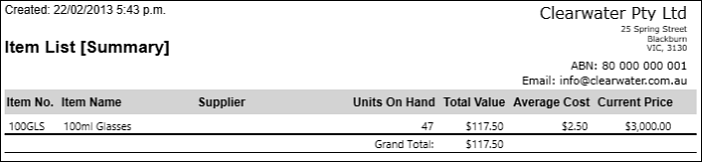Image Removed

Comparing the purchase debit note (shown below) with the Items List [Summary] report, you can see that if the debit note was recorded, the quantity of glasses would drop to negative 3, and the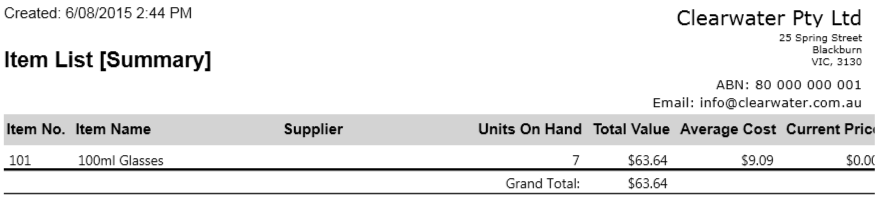Image Added

The list shows that the total value of the glasses is \$63.64. If the return was recorded, the total value of the glasses would drop to negative \$0.75. As both these values are less than zero, the software will prevent the purchase debit note from being recorded.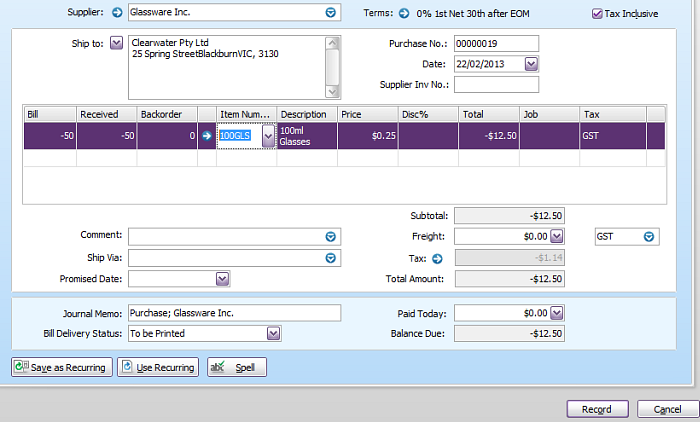Image Removed

From the comparison, the necessary adjustments can be calculated:

The quantity

below zero (-\$6.36), even though the quantity would be zero.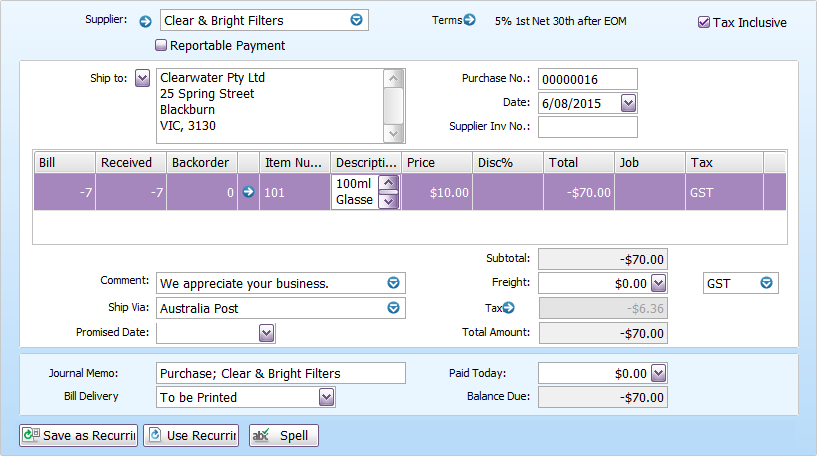Image Added

This comparison shows that the total value needs to be increased by

3 glasses, andThe total value

\$6.36, so an inventory adjustment needs to be

increased by \$0

done.

75

UI Expand
title 3. Make an Inventory Adjustment

An inventory adjustment is used to increase the quantity/ dollar value of the items. The amounts will be that of the previous task.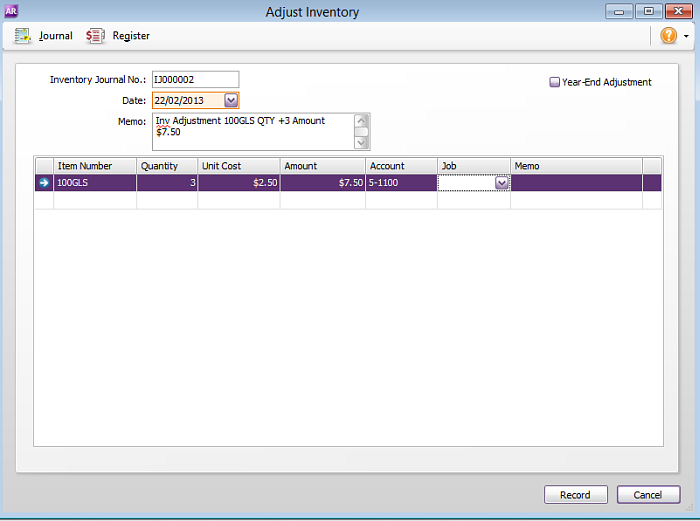Image Removed

HTML
`<h2><i class="fa fa-comments"></i>&nbsp;&nbsp;FAQs</h2><br>`
UI Expand
title Why did I get the negative inventory/non-zero value error?

You will get the negative inventory balance alert if any of these transactions have occurred:

• An item type purchase debit note (negative purchase) which exceeds either or both the quantity/dollar value of an inventoried item.
• Editing, reversing, or deleting a recorded item purchase which exceeds either or both the quantity/dollar value of an inventoried item.
• Editing, reversing or deleting an item type sale credit note (negative sale). This will remove items from inventory, and as such, the credit note must not remove more quantity/dollar value than is on hand.
• An Inventory Adjustment that attempts to adjust an item's quantity/dollar value to less than zero.
• An Inventory Transfer which attempts to adjust an item's quantity/dollar value to less than zero.
UI Expand
title What is the average costing method of inventory?

AccountRight uses the average costing method of inventory, which values your individual inventory based on the average unit price. The formula for calculating an item's average cost is:

Average Cost = Total Value of the item divided by the Total Quantity of the items

For example, a purchase of 10 items for \$10.00 (tax exclusive) will result in an average cost of \$10.00 per item. If the following day another 10 items were purchased for \$12.00 (tax exclusive), then:

• The total quantity of the items on hand = 20 items.

• The total value of the items = \$ 220.00.

• The average cost of the items = \$11.00 (\$220.00/20)

Complications can sometimes occur if differently priced items are taken out of the inventory and others are returned, while the item's average cost would remain the same.

In the example above, say after the second purchase, 10 items were sold, the 10 remaining items would retain their average cost of \$11.00 (\$110.00 Total Value). If the remaining 10 items were to be returned to the supplier at a price of \$12.00 each (\$120.00 Total Value), then the debit purchase note will result in a:

• zero quantity, and
• a less than zero dollar value.

Because of the less than zero value, the negative inventory error will be displayed. To correct this, an inventory adjustment will first be needed to increase the Total Value of the items. The inventory adjustment will show zero quantity, a zero unit cost and a positive \$10.00 in the amount column.

 Keep the quantity of the items at 0, because we don't want to add any more into the inventory. You can also ignore the unit cost because it will adjust automatically when you record the adjustment.In the Amount column, enter the total value difference you found in the previous task. In our example, it was \$6.36. If in your case you need to reduce the total value, enter the amount as a negative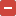.Finally, you'd enter the account you want to assign the adjustment amount to. This account is usually a cost of sales or inventory adjustment account.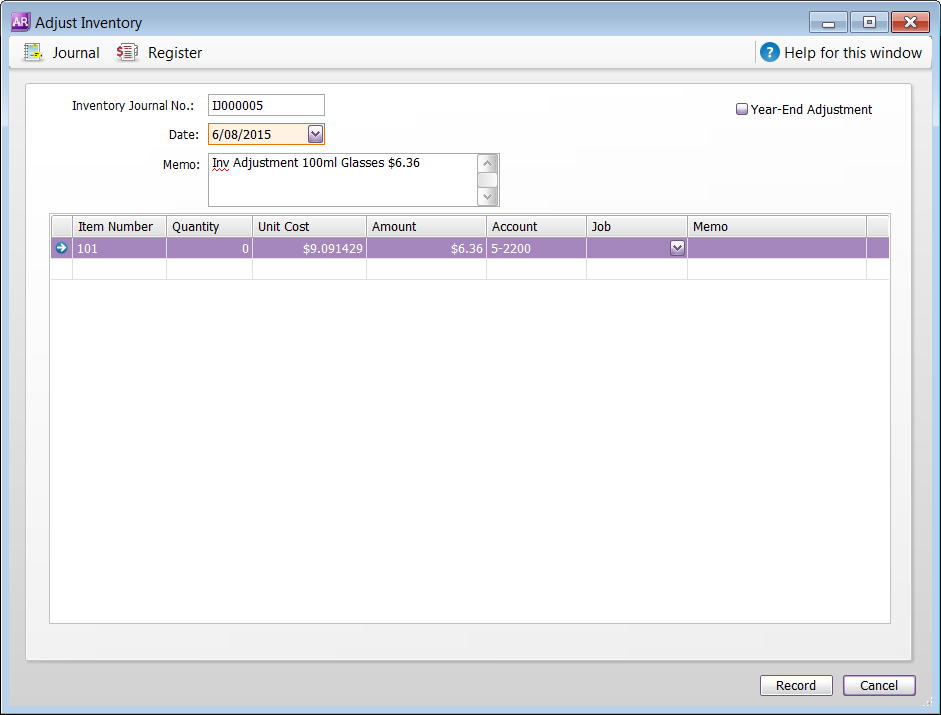Image Added
UI Text Box
type tip

Learn from the experts

Managing a full inventory can sometimes be tricky. The MYOB Inventory Training Course is an excellent resource to better understand AccountRight's inventory and its features - helping you stock smarter.

HTML
`</span>`
HTML-wrap
width 15% col span_1_of_5

HTML Wrap
float left col span_1_of_5
Panelbox
name green Related topics
Panelbox
name yellow From the community